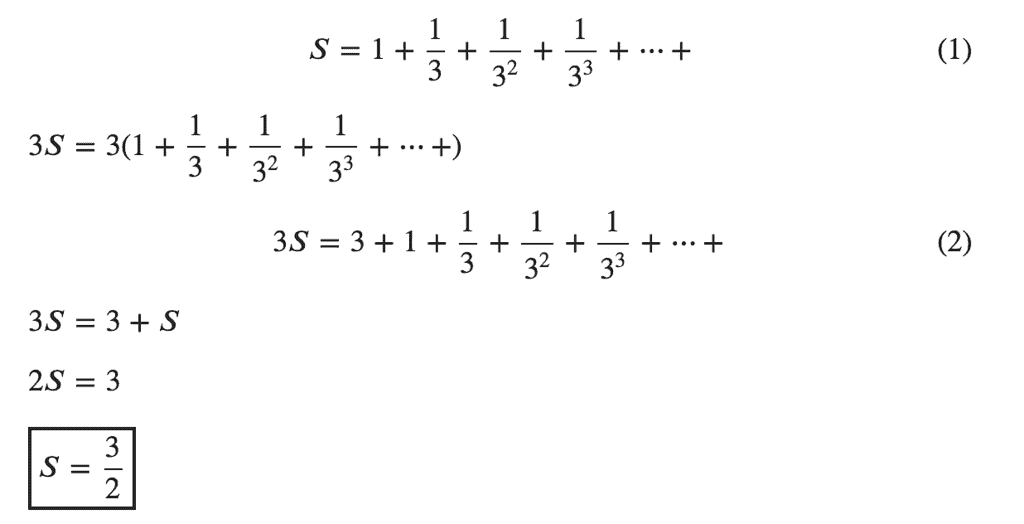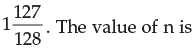# Test: Arithmetic And Geometric Progressions - 2

## 40 Questions MCQ Test Quantitative Aptitude for CA CPT | Test: Arithmetic And Geometric Progressions - 2

Description
Attempt Test: Arithmetic And Geometric Progressions - 2 | 40 questions in 40 minutes | Mock test for CA Foundation preparation | Free important questions MCQ to study Quantitative Aptitude for CA CPT for CA Foundation Exam | Download free PDF with solutions
QUESTION: 1

Solution:
QUESTION: 2

Solution:
QUESTION: 3

### Sum of the series 1 + 3 + 9 + 27 +….is 364. The number of terms is

Solution:
QUESTION: 4

The product of 3 numbers in G P is 729 and the sum of squares is 819. The numbers are

Solution:
QUESTION: 5

The sum of the series 1 + 2 + 4 + 8 + .. to n term

Solution:
QUESTION: 6

The sum of the infinite GP 14 – 2 + 2/7 – 2/49 + … is

Solution:
QUESTION: 7

The sum of the infinite G. P. 1 - 1/3 + 1/9 - 1/27 +... is

Solution:
QUESTION: 8

The number of terms to be taken so that 1 + 2 + 4 + 8 + will be 8191 is

Solution:

If you're talking about geometric series:

a = 1

r = 2/1 = 4/2 = 8/4 = 2

S(n) = a(1 - r^n) / (1 - r)

a(1 - r^n) / (1 - r) = 8191

1(1 - 2^n) / (1 - 2) = 8191

(1 - 2^n) / (-1) = 8191

1 - 2^n = 8191 * (-1)

1 - 2^n = -8191

-2^n = -8191 - 1

-2^n = -8192

2^n = 8192

n = log (base 2) of 8192

n = 13

QUESTION: 9

Four geometric means between 4 and 972 are

Solution:
QUESTION: 10

Three numbers are in AP and their sum is 21. If 1, 5, 15 are added to them respectively, they form a G. P. The numbers are

Solution:
QUESTION: 11

The sum of 1 + 1/3 + 1/32 + 1/33 + … + 1/3 n –1 is

Solution:QUESTION: 12

The sum of the infinite series 1 + 2/3 + 4/9 + .. is

Solution:
*Multiple options can be correct
QUESTION: 13

The sum of the first two terms of a G.P. is 5/3 and the sum to infinity of the series is 3. The common ratio is

Solution:
QUESTION: 14

If p, q and r are in A.P. and x, y, z are in G.P. then xq–r. y r–p. zp–q is equal to

Solution:
*Multiple options can be correct
QUESTION: 15

The sum of three numbers in G.P. is 70. If the two extremes by multiplied each by 4 and the mean by 5, the products are in AP. The numbers are

Solution:
*Multiple options can be correct
QUESTION: 16

The sum of 3 numbers in A.P. is 15. If 1, 4 and 19 be added to them respectively, the results are is G. P. The numbers are

Solution:
QUESTION: 17

Given x, y, z are in G.P. and xp = yq = zσ, then 1/p , 1/q, 1/σ are in

Solution:
QUESTION: 18

If the terms 2x, (x+10) and (3x+2) be in A.P., the value of x is

Solution:
QUESTION: 19

If A be the A.M. of two positive unequal quantities x and y and G be their G. M, then

Solution:
QUESTION: 20

The A.M. of two positive numbers is 40 and their G. M. is 24. The numbers are

Solution:
QUESTION: 21

Three numbers are in A.P. and their sum is 15. If 8, 6, 4 be added to them respectively, the numbers are in G.P. The numbers are

Solution:
QUESTION: 22

The sum of four numbers in G. P. is 60 and the A.M. of the 1st and the last is 18. The numbers are

Solution:
QUESTION: 23

A sum of Rs. 6240 is paid off in 30 instalments such that each instalment is Rs. 10 more than the proceeding installment. The value of the 1st instalment is

Solution:
QUESTION: 24

The sum of 1.03 + (1.03)2 + (1.03)3 + …. to n terms is

Solution:
QUESTION: 25

If x, y, z are in A.P. and x, y, (z + 1) are in G.P. then

Solution:
QUESTION: 26

The numbers x, 8, y  are in G.P. and the numbers x, y, –8 are in A.P. The value of x and y are

Solution:
QUESTION: 27

The nth term of the series 16, 8, 4,…. Is 1/217. The value of n is

Solution:
QUESTION: 28

The sum of n terms of a G.P. whose first terms 1 and the common ratio is 1/2 , is equal toSolution:
QUESTION: 29

t4 of a G.P. in x, t10 = y and t16 = z. Then

Solution:
QUESTION: 30

If x, y, z are in G.P., then

Solution:
QUESTION: 31

The sum of all odd numbers between 200 and 300 is

Solution:
QUESTION: 32

The sum of all natural numbers between 500 and 1000 which are divisible by 13, is

Solution:
QUESTION: 33

If unity is added to the sum of any number of terms of the A.P. 3, 5, 7, 9,…... the resulting sum is

Solution:
QUESTION: 34

The sum of all natural numbers from 100 to 300 which are exactly divisible by 4 or 5 is

Solution:
QUESTION: 35

The sum of all natural numbers from 100 to 300 which are exactly divisible by 4 and 5 is

Solution:
QUESTION: 36

A person pays Rs. 975 by monthly instalment each less then the former by Rs. 5. The first instalment is Rs. 100. The time by which the entire amount will be paid is

Solution:
QUESTION: 37

A person saved Rs. 16,500 in ten years. In each year after the first year he saved Rs. 100 more than he did in the preceding year. The amount of money he saved in the 1st year was

Solution:
QUESTION: 38

At 10% C.I. p.a., a sum of money accumulate to Rs. 9625 in 5 years. The sum invested initially is

Solution:
QUESTION: 39

The population of a country was 55 crose in 2005 and is growing at 2% p.a C.I. the population is the year 2015 is estimated as

Solution:
QUESTION: 40

If a b c are in A.P. as well as in G.P. then –

Solution:Use Code STAYHOME200 and get INR 200 additional OFF Use Coupon Code